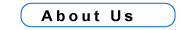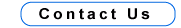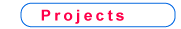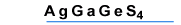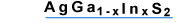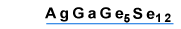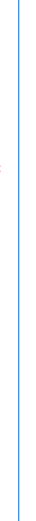AgGaGe5Se12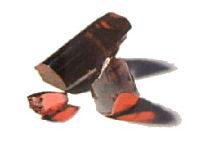Structural and Physical Properties
 Crystal symmetry and class Orthorhombic, C2v ( mm2 ) Space group C192v Cell parameters(A) a = 12.320, b = 23.64, c = 7.255 Melting point (oC) 713 ± 10 Optical homogeneity Dn < 1*10-4 Density (gram/cm3) 4.78 Absorption coefficient (cm -1) < 0.005Optical and NLO PropertiesOptical transmission ( mm ) 0.594 to 16.45 Fundamental absorption edge ( mm ) 0.56 Energy gap (eV) 2.2 Surface damage threshold ( t = 10 ns, l = 1.06 mm) - 50 MW/cm2Dispersion of refractive indices(Experimental values)l (mm) nx ny nz 1.064 2.4215 2.5683 2.5803
Nonlinear optical coefficients ( pm/V ):d31 = ± 28; d32 = ± 14.6; d33 = ± 14.6. X - c, Y - a, Z - b; At l = 1.064 mm 2V = 149.5o
dij defined in the abc frame (mm2 point group, c - polar axis)

Dispersion of refractive indices ( Sellmeier equations, l in mm )n2x = 5.7182737 - 24.729565 / ( 250.0 - l2 ) - 0.24365570 / ( 0.12550405 - l2 );
n2y = 6.5626531 - 116.75498 / ( 400.0 - l2 ) - 0.32805600 / ( 0.12928073 - l2 );
n2z = 6.6688973 - 136.03294 / ( 400.0 - l2 ) - 0.33109498 / ( 0.12679454 - l2 ).

Types of possible nonlinear processes in the principal planes
of the AgGaGe5Se12 and effective nonlinearity deff
 XY plane YZ plane XZ plane q < V oe - e, eo - e, deff = 0 oe - o, eo - o, deff = 0 oo - e, deff = d31 cosq; oo - e, deff = d32 sinj ee - o, deff = d32 sin2q + d31 cos2q oe - e, eo - e, deff = 0

Application

The AgGaGe5Se12 crystals can be used for frerquency doubling and three-frequency mixing. Parametric oscillators and amplifiers based of AgGaGe5Se12 can generate continuously tunable radiation in the spectral range from 1.2 to 15.6 mm with suitable pump laser.

 up about us contact us products